By going through these CBSE Class 11 Chemistry Notes Chapter 5 States of Matter, students can recall all the concepts quickly.

## States of Matter Notes Class 11 Chemistry Chapter 5

Most of the observable characteristics of chemical systems represent the bulk properties of matter. These are the properties associated with a collection of a large number of atoms, ions or molecules. For example, an individual molecule of a liquid does not boil, but the bulk boils.

Intermolecular Forces: Intermolecular forces are the forces of attraction and repulsion between interacting particles (atoms and molecules). This term does not include electrostatic forces that exist between the two oppositely charged ions and the forces that hold the atoms together in a molecule, i.e., a covalent bond.

Attractive intermolecular forces are known as van der Waals forces. They vary considerably in magnitude and include:

1. Dispersion or London forces
2. Dipole-dipole forces
3. Dipole-induced-dipole forces
4. A particularly strong type of dipole-dipole interaction is hydrogen bonding. But only a few elements (F, O, N) can participate in hydrogen bond formation.

Attractive forces between an ion and a dipole are known as ion-dipole forces and these are not van der Waals forces.

Dispersion Forces or London Forces: Atoms and non-polar molecules are electrically symmetrical and have no dipole moment because their electronic charge cloud is symmetrically distributed. But a dipole may develop momentarily even in such atoms and molecules. Suppose we have two atoms A and B close to each other [Fig. (a)]. It may so happen that momentarily electronic charge distribution in one of the atom A becomes unsymmetrical [Fig. (b) and (c)]. This results in the development of instantaneous dipole on atom A, for a very short time. This distorts the electron results a dipole is included in the atom.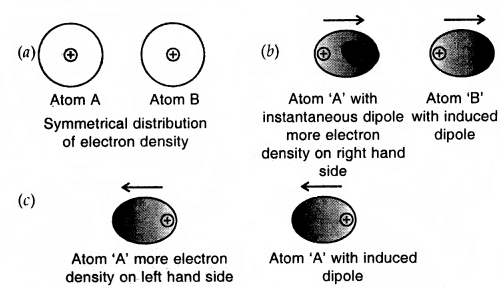Dispersion forces of London forces between atoms

The temporary dipole of atom ‘A’ and ‘B’ attract each other. Similarly, temporary dipoles are induced in molecules also. This force of attraction was first calculated by the German physicist Fritz London. For this reason force of attraction between two temporary dipoles is known as the London forces. Another name for this force is dispersion force.

These forces are always attractive and interaction energy is inversely proportional to the sixth power of the distance between two interacting particles (i.e., $$\frac{1}{r^{6}}$$ where r is the distance between two particles). These forces are important only at short distances (~ 500 pm) and the magnitude of the force depends on the polarisability of the particle. Dispersion forces are present among all particles.

Dipole-Dipole Forces:
Dipole-dipole forces act between molecules possessing permanent dipole. Ends of the dipole possess “partial charges” and these charges are shown by the Greek letter delta (5) Partial charges are always less than the unit charge (1.6 × 10-19 C) because of the electron sharing effect.

The polar molecules interact with neighbouring molecules. This interaction is weak compared to ion-ion interaction because only partial charges are involved. The interaction energy decreases with the increase of distance between the dipoles. It is proportional to $$\frac{1}{r^{6}}$$ where r is the distance between polar molecules. Besides dipole-dipole interaction, polar molecules can interact by London forces also. Thus the cumulative effect is that total of intermolecular forces in polar molecules increase. Fig. shows electron cloud distribution in the dipole of hydrogen chloride.(a) Distribution of electron cloud in HCl a polar molecule
(b) Dipole-dipole interaction between two HCl molecules

Dipole-Induced Dipole Forces
This type of attractive forces operates between polar molecules having permanent dipole and molecules lacking permanent dipole. The permanent dipole of the polar molecule induces dipole of the electrically neutral molecule by deforming its electronic cloud.

Thus induced dipole is developed in the other molecule. In this case, also interaction energy is proportional to -4 where r is the distance between two molecules Induced dipole moment depends UpOn the dipole moment present in the permanent dipole and the polarizability of the electrically neutral molecule.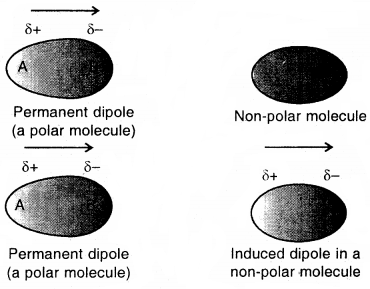Dipole-induced dipole interaction between a permanent dipole and induced dipole

In this case, the cumulative effect of dispersion forces and dipole- included dipole interactions exists.

Hydrogen Bond
The bond formed between the hydrogen atom of one molecule with the more electronegative atom (like N, O or F) of another molecule is called a hydrogen bond Such a molecule is highly polar. The electronegative atom of the covalent bond possesses lone pair of electrons. When two such molecules containing these type of bonds come close to each other, the hydrogen of one molecule is attracted towards the electronegative atom of the other molecule This interaction is represented by a dotted line and is called a hydrogen bond.

The energy of hydrogen bond varies between 10 to 100 kJ mol-1. This is a very significant amount of energy, therefore hydrogen bonds are a powerful force in determining the structure and properties of many compounds. The strength of the hydrogen bond is determined by the Coulomb interaction between the lone-pair electrons of the electronegative atom and the hydrogen atom. The following diagram shows the formation of the hydrogen bond.The intermolecular forces discussed so far are all attractive. Molecules also exert repulsive forces on one another. When two molecules are brought into close contact with each other, the repulsion between the electrons and between the nuclei in the molecules come into play. The magnitude of the repulsion rises very rapidly as the distance separating the molecules decreases. This is the reason that liquids and solids are hard to compress. In these states molecules are already in close contact, therefore they resist further compression.

→ Thermal Energy: Thermal energy is the energy of a body arising from the motion of its atoms or molecules. It is directly proportional to the temperature of the substance. It is the measure of the average kinetic energy of the particles of the matter and is thus responsible for the movement of particles. The movement of particles is called thermal motion.

Intermolecular Forces Vs Thermal Interactions
Intermolecular forces tend to keep molecules together but the thermal energy of the molecules tend to keep them apart. Three states of matter are the result of a balance between intermolecular forces and the thermal energy of the molecules.

The predominance of thermal energy and the molecular interaction energy of a substance in three states is depicted as follows: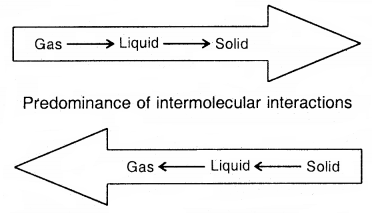The Gaseous State: Only 11 elements in the periodic table exist in the form of gases under normal conditions:

Gases are classified by the following properties.

1. Gases are the most compressible out of all the 3 states of matter.
2. Gases exert pressure equally in all directions.
3. Gases have a much lower density than solids and liquids.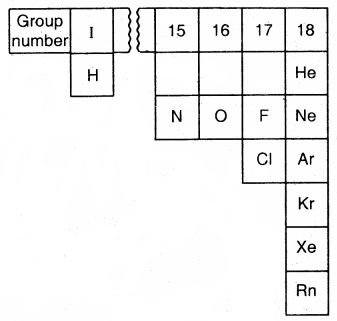elements that exist as gases
4. They assume the volume and shape of the container in which they are kept.
5. Gases mix evenly, and completely without any mechanical help.

Gas Law: Boyle’s Law (Pressure-Volume Relationship)
“Volume of a given mass of a gas is inversely proportional to its pressure provided the temperature is kept constant.”
V ∝ $$\frac{1}{P}$$ (mass and temperature kept constant)
or
PV = constant = k
or
P1V1 = P2V2, where P1V1 are the initial pressure and volume of the gas. P2 and V2 are the final values.

The value of k depends upon the amount of the gas, temperature of the gas, its nature and the units in which P and V are expressed.
Graphical Representation of Boyle’s Law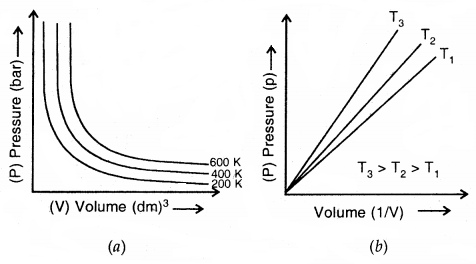(a) Graph of Pressure Vs Volume (b) Graph of pressure p Vs $$\frac{1}{V}$$

Each curve in (a) above corresponds to a different constant temperature and is known as an Isotherm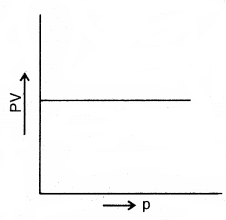(c) Graph of PV against P

1. A plot of P versus V at constant temperature for a fixed mass of gas would be a rectangular hyperbola (a)
2. A plot of P versus $$\frac{1}{v}$$ at constant temperature for a fixed mass of gas would be a straight line passing through the origin (b)
3. A plot of P versus PV at a constant temperature. In a fixed mass of a gas is a straight line parallel to the pressure axis:

Relationship between density and pressureCharle’s Law (Temp. Volume Relationship): The law states, “At constant pressure, the volume of a given mass of a gas increases or decreases by $$\frac{1}{273.15}$$ of its volume at 0°C and 1°C rise or fall in temperature.” Thus if the volume of the gas at 0°C and at t°C is Vo and Vt. Then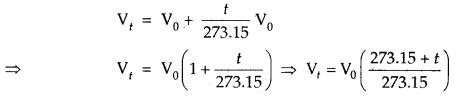At this stage, we define a new scale of temperature such that f°C on the new scale is given by T = 273.15 + t and 0°C will be given by To = 273.15. This new temperature scale is called the Kelvin temperature scale or Absolute temperature scale. It can be represented as follows: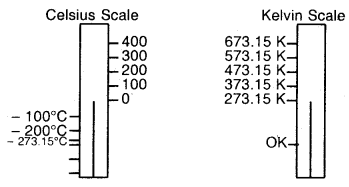Relationship between Kelvin Scale and Celsius Scale

One degree Celsius (1 °C) is equal to one Kelvin (K) in magnitude (Note that degree sign is not used while writing the temperature in absolute temperature scale, i.e. Kelvin scale). Only the position of zero has been shifted. Kelvin scale of temperature is also called the Thermodynamic scale of temperature and is used in all scientific works.

Thus we add 273 (more precisely 273.15) to Celsius temperature to obtain temperature at Kelvin scale.

A graph of volume Vs temperature at Kelvin scale will look like as shown.

If we write Tf = 273.15 + t and To = 273.15 in the equation we obtain the relationship
Vt = Vo($$\frac{\mathrm{T}_{t}}{\mathrm{~T}_{0}}$$)
⇒ $$\frac{V_{t}}{V_{0}}=\frac{T_{t}}{T_{0}}$$

Thus we can write a general equation as follows:
$$\frac{V_{2}}{V_{1}}=\frac{T_{2}}{T_{1}}$$
or
$$\frac{V}{T}$$ = constant = k

Each line of V vs T graph is called Isobar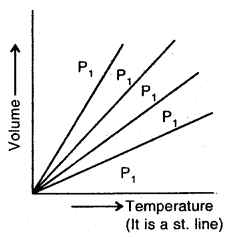At constant pressure for a given mass of a gas
V ∝ T
or
V = kT (where k is a constant, the value of k depends upon nature and amount of the gas)
or
$$\frac{V}{T}$$ = constant

If V1 = initial volume of the gas
T1 = initial temperature (absolute)
V2 = Final volume
T2 = Final temperature .
Then $$\frac{V_{1}}{T_{1}}=\frac{V_{2}}{T_{2}}$$ (mass and pressure kept constant)

Relationship between density and temperature
V ∝ T
or
$$\frac{1}{d}$$ ∝ T
or
dT = constant
or
d1T1 = d2T2

Gay-Lussac’s Law (Pressure-Temperature Relationship): It states that at constant volume, the pressure of a given mass of a gas varies directly with temperature on the Kelvin scale
Mathematically P ∝ T
It is also called Amonton’s Law
or
$$\frac{P}{T}$$ = constantPressure Vs Temperature (K) graph (Isometrics) of a gas

It states, “Equal volumes of all gases under similar conditions of temperature and pressure contain an equal number of molecules.”

Mathematically v ∝ n where n = no. of moles of the gas if T and P are constant.
n = $$\frac{m}{M}$$; where n = number of moles of gas
m = mass of the gas;
M = Molar mass of the gas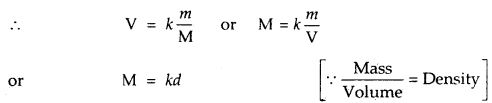where d is the density of the gas.

Thus the density of a gas is directly proportional to its formula mass.

Avogadro’s number or Avogadro’s constant is the number of particles present in 1 mole of a substance. This number is 6.023 × 1023.

One mole of each gas will have volume = 22.4 L at STP.

A gas that follows Boyle’s Law, Charle’s and Avogadro’s law strictly is called an ideal gas. Such gas is a hypothetical gas. It is assumed that intermolecular forces are not present between the molecules of an ideal gas. Real gases follow these laws only under certain specific conditions when forces of interaction are practically negligible. In all other situations, these deviate from ideal behaviour.

Ideal Gas Equation

1. At constant T and n, V ∝ $$\frac{1}{P}$$ Boyle’s Law
2. At constant P and n, V ∝ T Charle’s Law
3. At constant T and P, V ∝ n Avogadro’s Law
Thus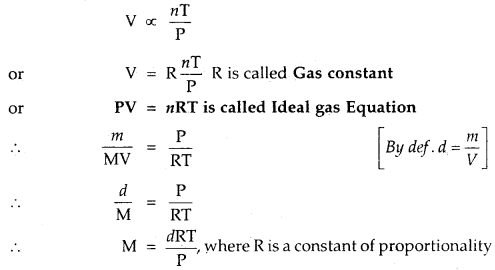On rearrangingPV= nRT … (1)

R is called Gas Constant. It is the same for all gases. Therefore, it is called Universal Gas Constant, equation (1) above is called Ideal Gas Equation.

The value of R for 1 Mole of an ideal gas can be calculated ai 273.15 K and 1 atm (1.013 × 105 Pascal) [Most of the real gases behave like ideal gases under these conditions) as follows.
R = $$\frac{\left(1.0325 \times 10^{5} \mathrm{~Pa}\right) \times\left(22.41383 \times 10^{-3} \mathrm{~m}^{3} / \mathrm{mol}\right)}{298.15 \mathrm{~K}}$$
= 8.31441 Pa m3 k-1 mol-1
= 8.3144 JK-1 mol-1

[For one mole V = 22.413832 for all gases at STP] For all practical purposes approximate value of R, i.e., 8.314 JK-1 mol-1 can be used.

In the equation PV = nRT

• P and T are Intensive properties. Intensive properties are independent of the ‘quantity’ or ‘bulk’ of the substance.
• n and V are Extensive properties. Extensive properties are dependent upon the ‘quantity’ or ‘bulk’ of the substance.

If temperature, volume and pressure of a fixed amount of the gas vary from T1, V1 and P1 to T2, V2 and P2.
Then $$\frac{\mathrm{P}_{1} \mathrm{~V}_{1}}{\mathrm{~T}_{1}}$$ = nR and $$\frac{\mathrm{P}_{2} \mathrm{~V}_{2}}{\mathrm{~T}_{2}}$$ = nR
or
$$\frac{P_{1} V_{1}}{T_{1}}=\frac{P_{2} V_{2}}{T_{2}}$$

It is called General Gas Equation or Combined gas law. Density and Molar Mass of a Gaseous substance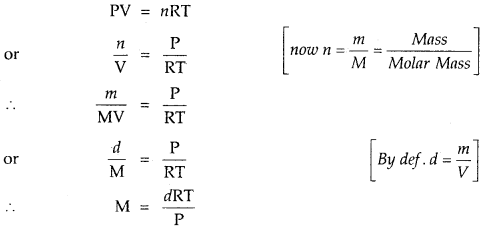Dalton’s Law of Partial Pressures: The law states, “Total pressure exerted by a mixture of non-reacting gases is equal to the sum of partial pressures exerted by the individual gases-all measurement being made at the same temperature.” Thus at constant temperature and volume:

PTotal = p1 + p2 + p3 + ………; PTotal = Total pressure.
p1, p2, …. are the partial pressures of the different non-reacting gases. ,

Gases are generally collected over water. The pressure of dry gas can be calculated by subtracting the vapour pressure of water from the moist gas which contains water vapours also. The pressure exerted by saturated water vapour is called aqueous tension.
Pdry gas = PTotal – Aqueous tension

Partial pressure in terms of mole fraction: Suppose three gases, enclosed in the volume V, exert partial pressure p1, p2 and p3 respectively. Then
p1 = $$\frac{n_{1} \mathrm{RT}}{\mathrm{V}}$$
p2 = $$\frac{n_{2} \mathrm{RT}}{\mathrm{V}}$$
p3 = $$\frac{n_{3} \mathrm{RT}}{\mathrm{V}}$$
where n1, n2 and n3 are number of moles of these gases. Thus expression for total pressure will be
PTotal = P1 + P2 + P3
= n1$$\frac{\mathrm{RT}}{\mathrm{V}}$$ + n2$$\frac{\mathrm{RT}}{\mathrm{V}}$$ + n3$$\frac{\mathrm{RT}}{\mathrm{V}}$$

= (n1 + n2 + n3)$$\frac{\mathrm{RT}}{\mathrm{V}}$$
On dividing p1 by PTotal we get
$$\frac{p_{1}}{P_{\text {Total }}}=\left(\frac{n}{n_{1}+n_{2}+n_{3}}\right) \frac{\mathrm{RTV}}{\mathrm{RTV}}=\frac{n_{1}}{n_{1}+n_{2}+n_{3}}$$ = x1

x1 is called mole fraction of first gas
Thus, p1 = x1 PTotal.

Similarly, for the other two gases, we can write
P2 = x2PTotal and P3 = x3PTotal

Thus a general equation can be written as
pi = xi PTotal

where pixi, and PTotalare partial pressure and mole fraction of with gas and total pressure of the gas mixture respectively. If the total pressure of the gases is known, the equation can be used to find out the pressure exerted by the individual gas.

Molar Volume of the gas under different conditions
(a) Standard temperature and pressure (STP) conditions are 0° C or 273,15 K and one atmospheric pressure. Under these conditions, 1 mole of the gas occupies a volume of 22.413996 L = 22.4 L or 22400 mL.

When STP conditions are taken as 0°C and 1 bar pressure [as 1 bar < 1 atom and 1 bar = 0.987 atm) molar volume is slightly higher and = 22.71098 L mol-1 = 22.7 L mol-1 or 22700 mL.

→ Standard Ambient Temperature and Pressure (SATP): Conditions are also used in some scientific works. SATP conditions mean 298.15 K and 1 bar (i.e. exactly 10s Pa). At SATP (1 bar and 298.15 K), the molar volume of an ideal gas is 22.789 mol-1 or 22800 mL.

→ Graham’s Law of Effusion/Diffusion: It states, “Under similar conditions of temperature and pressure, rate of diffusion of a gas is inversely proportional to the square root of the density of a gas.”

Mathematically Rd ∝ $$\sqrt{\frac{1}{d}}$$
Rd = Rate of diffusion, d = density of the gas
Rate of diffusion Rd = R$$\sqrt{\frac{1}{d}}$$

If two gases A and B diffuse under similar conditions of temperature and pressure rate rA and rB respectively and their densities are dA and dB respectively then
rA = k$$\sqrt{\frac{1}{d_{\mathrm{A}}}}$$
rB = k$$\sqrt{\frac{1}{d_{\mathrm{B}}}}$$

On dividing rA and rB we obtain
$$\frac{r_{\mathrm{A}}}{r_{\mathrm{B}}}=\sqrt{\frac{d_{\mathrm{B}}}{d_{\mathrm{A}}}}=\sqrt{\frac{d_{\mathrm{B}} \mathrm{V}}{d_{\mathrm{A}} \mathrm{V}}}=\sqrt{\frac{\mathrm{M}_{\mathrm{B}}}{\mathrm{M}_{\mathrm{A}}}}$$
where V is the volume of gas A and gas B which undergoes diffusion and MA and MB are molecular masses of gas A and gas B respectively. Rate of diffusion should be proportional to the average speed of the molecules of a gas. If average speed of molecules of gas A and gas B are nA and nB then
$$\frac{u_{\mathrm{A}}}{u_{\mathrm{B}}}=\sqrt{\frac{\mathrm{M}_{\mathrm{B}}}{\mathrm{M}_{\mathrm{A}}}}$$

Above equation can be used to determine the molar mass of gases.

→ Student’s Note: Though Graham’s Law of Diffusion is not included in the CBSE syllabus, but students are advised to go through it for various competitive examinations.

Kinetic Molecular Theory of Gases Postulates. Assumption of Kinetic Theory of Gases
1. Every gas is made up of a large number of extremely small particles called molecules. All the molecules of a particular gas are identical in mass and size and differ, in these from gas to gas.

2. The molecules of a gas are separated from each other by large distances so that the actual volume of the molecules is negligible as compared to the total volume of the gas.

3. The distances of separation between the molecules are so large that the forces of attraction or repulsion between them are negligible.

4. The force of gravitation on the molecules is also supposed to be negligible.

5. The molecules are supposed to be moving continuously in different directions with different velocities. Hence they keep on colliding with one another (called molecular collisions) as well as on the walls of the containing vessel.

6. The pressure exerted on the walls of the containing vessel is due to the bombardment of the molecules on the walls of the containing vessel.

7. The molecules are supposed to be perfectly elastic hard spheres so that no energy is wasted when the molecules collide with one another or with the walls of the vessel. The energy may, however, be transferred from some molecules to the other on collision.

8. Since the molecules are moving with different velocities, they possess different kinetic energies. However, the average kinetic energy of the molecules of a gas is directly proportional to the absolute temperature of the gas.

The behaviour of Real Gases
Deviation from ideal gas behaviour: A gas that obeys the ideal gas equation. (PV = nRT) at all temperatures and pressure is called Ideal Gas or Perfect Gas. Actually, none of the known gases obeys the ideal gas equation under all conditions of temperature and pressure. Such gases which do not obey the gas equation are called Real Gases.

However, most of the gases follow the ideal gas equation at low pressures and high temperatures. Appreciable deviation from the ideal gas behaviour is observed at low temperature and high pressure.

The temperature at which a real gas obeys the ideal gas law over an appreciable range of pressure is called Boyle Temperature or Boyle Point. Boyle point of a gas depends upon its nature.

A gas deviates from ideal behaviour when the product of the observed pressure and volume (P × V) is lower or higher than that expected from the ideal gas equation. Thus for a gas showing deviations from ideal behaviour (PV) observed < nRT
or
(PV)observed > nRT

Thus, to describe the behaviour of real gases, the ideal gas equation should be modified. This is conveniently done by inviting the ideal gas equation ‘in’ the form PV = Z(nRT).
or
Z = $$\frac{P V}{n R T}$$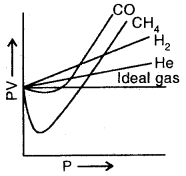The plot of PV Vs Pressure for real and ideal gas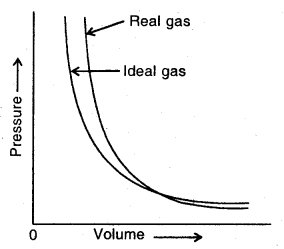The plot of Pressure Vs Volume for real gas and ideal gas

Where Z can have a value of one, less than one or more than one. The factor Z is called Compressibility Factor.
(a) When Z = 1, then PV = nRT, i.e., the gas shows an ideal gas behaviour. For an ideal gas Z = 1 under all conditions of temperature and pressure.
(b) When Z < 1, the observed PV value is less than the value for an ideal gas. Thus the gas shows a negative deviation when Z < 1.
(c) When Z > 1, the observed PV value is higher than that for an ideal gas. So when Z > 1, the gas shows positive deviations.

Equation of state for Real Gases (Van der Waal’s equation)
The van der Waal’s equation for one mole of a real gas is written
(P + $$\frac{a}{v^{2}}$$)(v-b) = RT
If n moles of a real gas is taken

The equation becomes
(P + $$\frac{a n^{2}}{v^{2}}$$)(v – b) = nRT
Here ‘a’ and ‘b’ are called van der Waals parameters or constants which vary from gas to gas. Their value depends upon the gas nature.

The cause of the deviation is actually due to two faulty assumptions of the kinetic theory
They are

• There is no force of attraction and repulsion between the molecules of a gas.
• The volume of the molecules of a gas is negligibly small as compared to the empty space between them.

Derivation of van der Waal’s equation
1. Correction for volume: Suppose the volume occupied by the gas molecules is v. When the molecules are moving, their effective volume is four times the actual volume i.e., 4v. Let us called it b i.e. b = 4v (called excluded volume of co-volume). Thus the free volume available to the gas molecules for movement i.e.

Corrected volume = (V – b) for one mole
= (V – rib) for n moles

2. Correction for pressure. A molecule (A) lying within the vessel is attracted equally by other molecules on all sides but a molecule near the wall (B) is attracted (pulled back) by the molecules inside (Fig.) Hence it exerts less pressure. In other words, the observed pressure is less than the ideal pressure. Hence

Corrected pressure = P + p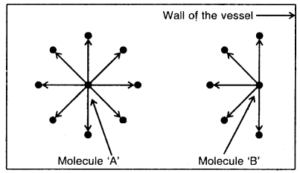Backward pull on molecule B by other molecules

Evidently, the correction term p is proportional to density of the gas near the wall and the density of the gas inside i.e.
p ∝ (density)2
or
p ∝ d2
But d ∝ $$\frac{1}{V}$$ for one mole
or
d ∝ $$\frac{1}{\mathrm{~V}^{2}}$$ for 1 mole
or
p = $$\frac{a}{\mathrm{~V}^{2}}$$
or
p ∝ $$\frac{n^{2}}{\mathrm{~V}^{2}}$$ for n moles
or
p = $$\frac{a n^{2}}{V^{2}}$$

∴ Corrected pressure = (P + $$\frac{a}{\mathrm{~V}^{2}}$$) for 1 mole
= (P + $$\frac{a n^{2}}{V^{2}}$$) for n moles
where a is constant depending upon the nature of the gas.

Substituting the correct values of volume and pressure in the ideal gas equation we get
(P + $$\frac{a}{\mathrm{~V}^{2}}$$)(V – b) = RT for 1 mole
or
(P + $$\frac{a n^{2}}{V^{2}}$$)(V – nb) = nRT for n moles

Significance of van der Waal’s constants
1. van der Waal’s constant ‘a’: Its value is a measure of the magnitude of the attractive forces among the molecules of the gas. The greater the value of ‘a’, the larger is the intermolecular forces of attraction.

2. van der Waal’s constant ‘b’: Its value is a measure of the effective size of the gas molecules. Its value is equal to four times the actual volume of the gas molecules. It is called excluded volume or co-volume.

Units of van der Waal’s constant
1. Units of ‘a’. As p = $$\frac{a n^{2}}{V^{2}}$$
∴ a = $$\frac{p \times V^{2}}{n^{2}}$$
= atm L2 mol2 or bar dm6 mol-2

2. Units of ‘b’. As volume correction
v = nb,
∴ b = $$\frac{v}{n}$$ = K mol-1dm3mol-1.

Explanation of the behaviour of Real gases by van der Waal’s equation
1. At very low pressure, V is very large. Hence the correction term a.V2 is so small that it can also be neglected in comparison to V. Thus van der Waal’s equation reduces to the form PV = RT. This explains why at very low pressures, the real gases behave like ideal gases.

2, At moderate pressures, V decreases. Hence a/V2 increases and cannot be neglected. However, V is still large enough in comparison to ‘b’ so that ’b’ can be neglected. Thus van der Waal’s equation becomes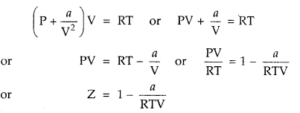Thus compressibility factor is less than 1. As pressure is increased at a constant temperature, V decreases so that the factor n/RTV increases. This explains why initially a dip in the plot of Z versus P is observed.

3. AT high pressures, V is so small that ‘b’ cannot be neglected in comparison to V. The factor a/V2 is no doubt large but as P is very high, a/V2 can be neglected in comparison to P. Thus van der Waal’s equation reduces to the form
P(V -b) = RT
or
PV = RT + Pb ⇒ $$\frac{\mathrm{PV}}{\mathrm{RT}}$$ = 1 + $$\frac{\mathrm{P} b}{\mathrm{RT}}$$
or
Z = 1 + $$\frac{\mathrm{P} b}{\mathrm{RT}}$$

Thus compressibility factor is greater than 1. As P is increased (at constant T), the factor Pb/RT increases. This explains why after minima in the curves, the compressibility fact of increases continuously with pressure.

4. At high temperatures, V is very large (at a given pressure) so that both the correction factors (a/V2 and b) become negligible as in case (i). Hence at high temperature, real gases behave like an ideal gas.

→ Explanation of the exceptional behaviour of hydrogen and helium may be seen that for H2 and He, the compressibility factor Z is always greater than l and increases with the increase of pressure: This is because H2 and He being very small molecules, the intermolecular forces of attraction in them are negligible i.e., V is very very small so that a/V2 is negligible
P(V -b) = RT
or
PV = RT + Pb
or
$$\frac{\mathrm{PV}}{\mathrm{RT}}$$ = 1 + $$\frac{\mathrm{PB}}{\mathrm{RT}}$$

Thus, $$\frac{\mathrm{PV}}{\mathrm{RT}}$$ i.e., Z > 1 and increases with increase in the value of P at constant.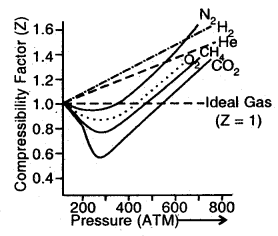(a) Z vs P for different gases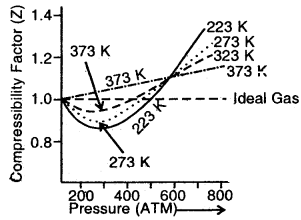(b) Z as P to N2 gas at different temperatures

Plots in Fig. (b) show that as the temperature increases, the minimum in the curve shifts upwards. Ultimately, a temperature is reached at which the value of Z remains close to 1 over an appreciable range of pressure. For example, in the case of N2 at 323 K, the value of Z remains close to 1 up to nearly 100 atmospheres.

For a real gas Z = $$\frac{p V_{\text {real }}}{n \mathrm{RT}}$$ ……….(1)
If the gas shows ideal behaviour then
V ideal = $$\frac{nRT}{p}$$
On putting the value of $$\frac{nRT}{p}$$ in the above equation (1)
Z = $$\frac{\mathrm{V}_{\text {real }}}{\mathrm{V}_{\text {ideal }}}$$

Thus compressibility factor may also be defined as the ratio of actual molar volume of a gas to the molar volume of it if it were ideal at that temperature and pressure.

→ Liquefaction of Gases and Critical Point: At high pressures and lower temperatures deviation from ideal behaviour for gases is observed. At high pressures, molecules of the gas come closer and attractive forces start operating. As the temperature is lowered further, the attractive forces draw the molecules together to form a ‘liquid’. This temperature is called liquefaction temperature. It depends upon the nature of the gas and its pressure.

The critical temperature for gas is that temperature above which it is not possible to liquefy it, however large is the pressure applied on it. CO2 gas remains a gas even when a pressure of 73 atmospheres is applied to it. However at this pressure when it is cooled to 30.98°C, it starts liquifying. So for CO2 30.98°C is its critical temperature. The volume of a gas at critical temperature is called its critical volume and pressure (73 atm. for CO2) is critical pressure.

Critical pressure is the pressure required to liquefy the gas at the critical temperature. All three of them are collectively called critical constants of the gas and are represented by Tc, Pc and Vc. For example, critical constants of CO2 are:
Tc = 31.1°C, Pc = 73.9 atm, Vc = 95.6 cm3 mol-1Isotherms of CO2 (From Andrew’s experiment)

At the lowest temperature employed i.e., 13.1°C, at low pressure. CO2 exists as a gas, as shown at point A. As the pressure is increased, the volume of the gas starts. Hence volume decreases rapidly along with BC because the liquid has much less volume than gas. At point C, liquefaction is complete. Now the increase in pressure has very little effect upon volume because liquids are very little compressible.

Hence a step curve CD is obtained. As the temperature is increased, the horizontal portion becomes smaller and smaller and at 31.1°C it is reduced at a point, P. This means that above 31.1°C, the gas cannot be liquefied at all, however, high pressure may be applied. Thus 31.1°C, is the critical temperature. The corresponding pressure to liquefy the gas at the critical temperature is its critical pressure, P (i.e., 73.9 atm). The volume occupied by 1 mole of the gas under these conditions is its critical volume, V. (i.e., 95.6 ml).

→ Liquid State: In liquids, intermolecular forces of attraction are much large than in gases. Unlike gases, liquids have a definite volume, but no definite shape. The molecules in a liquid are in constant random motion. The average kinetic energy of the molecules in a liquid is proportional to absolute temperature.

→ Vapour Pressure: Vapour pressure of a liquid at any temperature is the pressure exerted by the vapour present above the liquid in equilibrium with the liquid. The magnitude of vapour pressure depends upon the nature of, liquid and temperature. At equilibrium between the liquid and the vapour phase, the vapour pressure becomes steady or constant and at this stage, it is called Equilibrium Vapour Pressure or saturated vapour pressure.

The Boiling Point of a liquid is the temperature at which its vapour pressure becomes equal to external pressure. At 1 atm pressure boiling temperature is called the normal boiling point. If pressure is one bar, then the boiling point is called standard boiling point standard boiling point of a liquid is slightly lower than the normal boiling point (because 1 bar < 1 atm)

The normal boiling point of water is 100°C (373 K) and its standard boiling point is 99.6°C (373.6 K).

The Vapour pressure of a liquid increases with an increase in temperature, with more molecules of the liquid going into the vapour phase, the liquid becomes less dense and the density of the vapour phase increases. When the density of the liquid and vapours becomes the same the clear boundary between the liquid and the vapour disappears. This temperature is called the critical temperature of the liquid.

→ Surface Tension: The surface tension of a liquid is defined as the tangential force acting along the surface of a liquid at right angles along one unit length drew on the surface of the liquid.

It is the work done to increase the free surface area of any liquid by one unit at constant temperature and pressure.

The S.I. unit of surface tension is Nm-1. The C.G.S. unit of surface tension is dyne-1 cm-1. The lowest energy state of the liquid will be when its surface area is minimum. Spherical shape satisfies this condition. Hence raindrops or mercury drops are spherical in shape. Liquids tend to rise in the capillary due to surface tension. Surface tension decreases as the temperature is raised. It has dimensions of kg s-2. In terms of surface energy per unit area, its dimensions are Jm-2. ,

→ Viscosity: Viscosity is a measure of resistance that arise due to the internal friction between layers of fluid as they slip past one another. It is the internal resistance to the flow of liquid. The liquids which flow rapidly have low internal resistance and hence are said to be less viscous, i.e., their viscosity is low. On the other hand, the liquids which flow slowly have high internal resistance and hence are said to be more viscous, i.e., their viscosity is high The viscosity of honey or glycerol is higher than, say, water.

The coefficient of viscosity is defined as the force applied per unit area which will maintain a unit relative velocity between the two layers of a liquid at unit distance from each other. It is represented by q. Liquids having low q values are called mobile while those having high q value are termed as viscous.

If f is the force required to maintain the flow of layers, then
f ∝ A [A is the area of contact]
f ∝ $$\frac{d u}{d Z}$$
or
f = ηA$$\frac{d u}{d Z}$$; η is called coefficient of viscosity.

S.I. unit of viscosity coefficient is 1 newton second per square metre [Nsm-2]

In the CGS system, its unit is poise
1 poise = 1 gm cm-1 s-1 = 10-1 kg m-1 s-1

Viscosity decreases with an increase in temperature. Hydrogen bonding and van der Waal’s forces are strong enough to cause High viscosity.

→ van der Waals forces: Attractive intermolecular forces between interacting particles (atoms and molecules) are called van der Waals forces.

→ Dispersion Force/London Force: Atoms and non-polar molecules are electrically symmetrical and hence no dipole moment. But a dipole may develop momentarily even in such atoms and molecules. This force of attraction between two temporary dipoles is called the London force or dispersion force.

→ Dipole-Dipole Forces: Dipole-dipole forces act between the molecules possessing permanent dipole. The polar molecules interact with neighbouring molecules as in the case of HCl molecules.

→ Dipole-induced Dipole Forces: This type of attractive forces operate between the polar molecules having permanent dipole and the molecules lacking permanent dipole. The permanent dipole of the polar molecule induces dipole on the electrically neutral molecule by deforming its electronic cloud Thus an induced dipole is developed in the other molecule.

→ Hydrogen Bond: It is a special case of dipole-dipole interaction It is present in molecules having H-atom attached to a small-sized highly electronegative atom like F, O, N. The highly electronegative attracts H-atom of the neighbouring molecule through electrostatic force of attraction It is a weak bond and present in molecules like I IF, FRO, R-OH etc.

→ Thermal Energy: It is the energy of a body arising due to the motion of its atoms or molecules.

→ Boyle’s Law: At constant temperature, the volume of a given mass of a gas is inversely proportional to its pressure.

→ Charles’ Law: Pressure remaining constant, the volume of a given mass of a gas is directly proportional to its absolute temperature.

→ Isotherm: Each curve obtained on plotting different values of, pressure against volume at constant temperature is called Isotherm.

→ Isobar: Each line of the volume vs temperature graph at constant j pressure is called Isobar.

→ Kelvin temperature/Absolute temperature scale. It is obtained by adding 273.15 to the temperature on the Celsius scale.
Kelvin-Tem. = T = t°C + 273.15. It is also called the Thermodynamic ’ scale.

→ Absolute zero: The volume of all gases becomes zero at – 273.15°C. The lowest hypothetical or imaginary temperature at which gases cease to exist is called absolute zero.

→ Gay-Lussac’s Law: At constant volume, the pressure of a given j mass of a gas varies directly with the pressure.

→ Isochore: Each line of the graph obtained by plotting pressure vs Kelvin temperature at constant volume is called.Isochore.

→ Avogadro’s Law: Equal volumes of all gases under the similar condition of temperature and pressure contain an equal number of molecules.

→ Avogadro’s constant: The number of molecules in one mole of a gas is – 6.022 × 1023 and the number 6.022 × 1023 is called Avogadro’s constant.

→ Ideal Gas Equation.
PV = nRT
where P = Pressure,
V = volume,
R = Universal gas constant
n = no. of moles,
T = absolute temperature

For 1 mole of an ideal gas PV = RT.

Combined gas law $$\frac{\mathrm{P}_{1} \mathrm{~V}_{1}}{\mathrm{~T}_{1}}=\frac{\mathrm{P}_{2} \mathrm{~V}_{2}}{\mathrm{~T}_{2}}$$

→ Dalton’s Law of Partial Pressures: The total pressure exerted by the mixture of non-reactive gases is equal to the sum of partial pressures exerted by individual gases under similar conditions of temperature and volume.

→ Aqueous Tension: Pressure exerted by the saturated water vapour is called Aqueous Tension. ,

→ Compressibility Factor: The deviation of a gas from ideal behaviour can be measured in terms of compressibility factor Z which is the ratio of product pV and nRT.
Z = $$\frac{pV}{nRT}$$

→ Boyle Point or Boyle Temperature: The temperature at which a real gas obeys the ideal gas law is called Boyle point/temperature.

→ Critical Temperature: The temperature above which a gas cannot be liquified, however large may be the pressure applied on it.
Or
It is a temperature below which a gas can be liquified with the application of pressure is called its critical temperature (Tc) Critical Pressure: The pressure required to liquefy a gas at its critical temperature is called its critical pressure (Pc).

→ Critical Volume: The volume possessed by gas at its critical temperature is called its critical volume (Vc).

→ Boiling Point: It is a temperature at which the vapour pressure of a liquid becomes equal to external pressure.

→ Normal Boiling Point: At 1 atmospheric pressure, the boiling temperature of a liquid is called its normal boiling point.

→ Standard Boiling Point: If the pressure is 1 bar, the boiling temperature of the liquid is called standard boiling point, the standard boiling point of a liquid is slightly lower than the normal boiling point (as 1 bar is slightly < 1 atm).

→ Surface Tension: It is defined as the force acting per unit length perpendicular to the line drawn on the surface of the liquid.

→ Unit: Surface Tension (γ-Gamma) in S.I. scale = Nm-1 V Its dimensions are kgs-2.

→ Viscosity: It is a measure of the resistance of the flow of a liquid which arises due to internal friction between layers of it as they slip past one another while liquid flows.

Important Formulae
→ Boyle’s Law: At T constant
V ∝ $$\frac{1}{P}$$
or
PV = constant
If P1, V1 are the initial pressure, the volume of a gas at constant temperature and P2, V2 are its final pressure and volume respectively.
then P1V1 = P2V2

→ Charles’ Law: At constant pressure
V ∝ T, where T is the absolute temperature
or
$$\frac{V}{T}$$ = Constant
or
$$\frac{\mathrm{V}_{1}}{\mathrm{~T}_{1}}=\frac{\mathrm{V}_{2}}{\mathrm{~T}_{2}}$$
or
$$\frac{\mathrm{V}_{1}}{\mathrm{~V}_{2}}=\frac{\mathrm{T}_{1}}{\mathrm{~T}_{2}}$$
Gay Lassac’s Law : P ∝ T if volume is kept constant
or
$$\frac{P}{T}$$ = constant

→ Ideal Gas Equation:
PV = nRT

→ Combined Gas Law:
$$\frac{P_{1} V_{1}}{T_{1}}=\frac{P_{2} V_{2}}{T_{2}}$$

→ Coefficient of Viscosity : If the velocity of the layer at a distance dZ is changed by a value du, then velocity gradient is given by the amount $$\frac{d u}{d Z}$$. The force required lo maintain the flow of layers is proportional to the area of contact of layers and velocity gradient,
i.e., f ∝ A [A is the area of contact]
f ∝ $$\frac{d u}{d Z}$$
∴ f ∝ A$$\frac{d u}{d Z}$$
or
f = ηA$$\frac{d u}{d Z}$$

where η is proportionality constant called coefficient of viscosity.
If A = 1 $$\frac{d u}{d Z}$$ = 1
then f = η
Therefore, the Coefficient of viscosity is defined as the force when the velocity gradient is unity and the area of contact is unity.

SI unit of viscosity coefficient is 1 newton sec m-2
= Ns m-2 = pascal second
1 Pa s = 1 kg m-1 s-1

In CGS system coefficient of viscosity is poise
1 poise = 1 g cm-1 s-1 = 10-1 kg m-1 s-1.